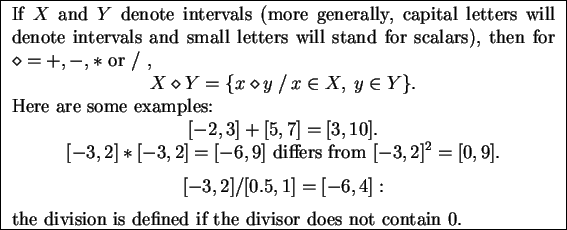### Software

• MPFI, a multiple precision interval arithmetic library based on MPFR
• MPFI stands for Multiple Precision Floating-point Interval library.

The basic principle of interval arithmetic consists in enclosing every number by an interval containing it and being representable by machine numbers: for instance it can be stored as its lower and upper endpoints and these bounds are machine numbers, or as a centre and a radius which are machine numbers. For example, on a radix-10 machine with 3 digits of mantissa,would be represented by the interval [3.14, 3.15]. The arithmetic operations are extended for interval operands in such a way that the exact result of the operation belongs to the computed interval.When operands are replaced by intervals in an expression, such as a polynomial one, the computed result is an interval enclosing every possible value of this expression for any scalar value in the input intervals: this is called guaranteed result and is the main advantage of interval arithmetic. However, this result can be too large and depends on the chosen form for the evaluated expression.

The two main origins of this overestimation of the results are on the one hand the decorrelation of the variables, also known as dependency problem: when computing X*X, what is computed is { x*y / x in X, y in X } and the correlation (identity) between x and y is lost; and on the other hand the wrapping effect: in 2 (or more) dimensions, the result must be a rectangular box with sides parallel to the axes, enclosing an oblique and possibly very thin set.

Thanks to the following result, it is possible to circumvent this difficulty: it has been proven that for a large class of functions called arithmetic expressions by Neumaier (see references), the width of a function enclosure is proportional to the width of the input interval if ideal (exact) arithmetic is used. This theorem has been extended to take into account rounding errors. This class of functions can roughly be described as functions defined without involving the endpoints of their arguments and without tests.

A first solution thus consists in splitting the input intervals into small subintervals before evaluating the function on each subinterval and taking the union of these partial results, thus paving the resulting set. The use of Taylor of order 1 or 2 is also classical in order to overcome the swell of the resulting intervals: they provide sharper bounds than other evaluations when the input interval is tight and larger ones otherwise.

These two ways of sharpening the computed intervals require the ability to split an input interval into small subintervals and possibly very thin ones. This need has motivated the use of an arbitrary precision arithmetic as the underlying arithmetic for our interval library. A second requirement for interval arithmetic is the outward rounding functionality: it is provided by MPFR, the underlying multiple precision arithmetic library of MPFI.

For further motivations and explanations on the need for an arbitrary precision interval arithmetic library, see also the paper by N. Revol and F. Rouillier, Motivations for an arbitrary precision interval arithmetic and the MPFI library, published in Reliable Computing, vol. 11, no 4, pp 275--290, 2005.

MPFI is intended to be a portable library written in C for arbitrary precision interval arithmetic with intervals represented using MPFR reliable floating-point numbers. It is based on the GNU MP library and on the MPFR library. The purpose of an arbitrary precision interval arithmetic is on the one hand to get guaranteed results, thanks to interval computation, and on the other hand to obtain accurate results, thanks to multiple precision arithmetic. The MPFI library is built upon MPFR in order to benefit from the correct roundings provided by MPFR. Further advantages of using MPFR are its portability and compliance with the IEEE 754 standard for floating-point arithmetic. This version of MPFR/MPFI is released under the GNU Library General Public License.

You can download MPFI 1.5.4, the latest release of MPFI: mpfi-1.5.4.tar.bz2 mpfi-1.5.4.tar.gz mpfi-1.5.4.tar.xz mpfi-1.5.4.zip.
Since MPFI is based on MPFR which is built upon GMP, you need to have installed GMP and the CVS version of MPFR.

You can still download the older and deprecated version of MPFI 1.0 (last revised 2002-04-19), based on MPFR version 2.0.1 or higher, and GMP version 4.0 or higher, at your own risks.

This is a largely lacunary list of introductory references.

• G. Alefeld and J. Herzberger, Introduction to interval analysis, Academic Press, 1983.
• R. B. Kearfott, Rigorous global search: continuous problems, Kluwer, 1996.
• E. Hansen, Global optimization using interval analysis, Marcel Dekker, 1992.
• R. Moore, R. B. Kearfott, M. J. Cloud, Introduction to Interval Analysis, SIAM 2009.
• A. Neumaier, Interval methods for systems of equations, Cambridge University Press, 1990.
• H. Ratschek and J. Rokne, New computer methods for global optimization, Ellis Horwood Ltd, 1988.

This is the main Web site for interval computations.

MPFI is a joint work with Fabrice Rouillier.